Expert Modelling: Hands-on help from Rocscience Engineers. Register Here

# Define Hydraulic Properties

The Define Hydraulic Properties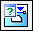option, located in the toolbar or by selecting Properties > Define Material Properties, is used to specify the groundwater/hydraulic parameters for each material. Define Hydraulic Properties is only applicable if you are considering pore pressure in your RS2 analysis (i.e. using Piezometric Lines, Water Pressure Grids, Ru values, or finite element seepage analysis). If you are NOT considering pore pressure in your analysis, then Define Hydraulic Properties is not applicable.

The options available in the Define Hydraulic Properties dialog correspond to the Groundwater Method specified in the Project Settings dialog:

• If the Groundwater Method = Finite Element Analysis, then you will be able to define the saturated and unsaturated permeability characteristics of each material.

Note: Piezometric Lines and Grids can be defined when any Groundwater Method is selected.

If the Groundwater Method = Finite Element Analysis, see the Hydraulic Properties (Seepage Analysis) topic for information about the input parameters.

Note:

• The materials in the Define Hydraulic Properties dialog correspond to the materials in the Define Material Properties dialog (i.e. the dialog in which you define the strength properties of your materials).
• The material names and colours which appear in the Define Hydraulic Properties dialog are those which are specified in the Define Material Properties dialog. The names and colours CANNOT be edited in the Define Hydraulic Properties dialog -- if you wish to edit the name or colour of a material, this must be done in the Define Material Properties dialog.

## Piezo to Use

Piezometric Lines are created with the with the Add Piezometric Line option. Each Piezometric Line has an ID number associated with it. These ID numbers are automatically created by RS2, when the Piezometric Line is added to the model.

• In order to assign a Piezometric Line to a given material, select the ID number of the desired Piezometric Line from the Piezo to Use drop-list. The selected Piezometric Line will then be used for the pore pressure calculations for that material.
• If you want to specify zero pore pressure for the material then select Piezo to Use = None.
• If Piezo to Use = None, you can specify an Ru value to calculate pore pressure for the material, see below.

Note: the Piezo to Use option is applicable if you want to use ONE Piezometric Line for the pore pressure calculation for a given material, AT ALL STAGES OF THE MODEL (for staged models). If you require different piezo lines to be applied at different stages, then use the Stage Piezo Lines option described below.

## Stage Piezo Lines

If your model consists of multiple stages, and you require different piezo lines to be applied at different stages, for a given material, then use the Stage Piezo Lines option. This allows you to simulate, for example, the draw down of a water table at different stages.

To assign the staging of Piezometric Lines:

1. In the Define Hydraulic Properties dialog, select the Stage Piezo Lines check box.
2. You will notice that the Piezo to Use option becomes disabled, and the area below the Stage Piezo Lines check box becomes enabled.
3. Now select the Add Stage button, until the number of rows is equal to the number of stages at which you need to specify a Piezometric Line. For example:
• If you need to assign 3 Piezometric Lines at 3 different stages, then select Add Stage TWICE, so that a total of 3 rows appears in the data entry area below the Stage Piezo Lines check box.

Note: if the Add Stage button is disabled, this means that you have not set the Number of Stages in Project Settings. In this case, go to the Project Settings dialog, and define the number of stages.

1. In each row, enter the Stage number at which the Piezometric Lines are to be assigned.
2. In each row, select the Piezo ID number representing the Piezometric Line that you wish to assign at the specified stage.
3. It is important to note that you only need to define the Stage and Piezo ID information, for the stages at which the Piezometric Line will change. You DO NOT need to define this information at stages where the Piezometric Line does not change.
4. If you accidentally add too many rows with the Add Stage button, click the mouse in a row and select the Delete Stage button, to delete the row.
5. When you are finished entering the Stage and Piezo ID numbers, if your model consists of multiple materials with the same staging of Piezo Lines, you can use the Copy To button to automatically copy the Stage / Piezo ID information to other materials in the dialog (see below).
6. When the analysis is run, RS2 will use the Stage and Piezo ID information in the Define Hydraulic Properties dialog, to determine the correct Piezometric Line to be used at each stage of the analysis, for each material.

Note: you can use the View Piezos by Stage option to view only the piezo line(s) which are applicable for the current stage. This allows you to easily verify that the correct staging sequence of the piezometric lines has been applied.

## Hu Value

The Hu value is simply a factor between 0 and 1, by which the vertical distance from a point (in the soil or rock), to a Water Surface (i.e. Piezo Line) is multiplied to obtain the pressure head. The Hu value is used to calculate pore pressure as follows:

u = ywhHu

where:

u = pore pressure

yw = the Pore Fluid Unit Weight (entered in the Project Settings dialog)

h = the vertical distance from a point to a Piezometric Line

Hu = the Hu value for the material

Note: if the distance h is negative, (i.e. a point is above the Piezometric Line) then the pore pressure is set to zero at that point.

There are two ways of defining the Hu value - Auto or Custom.

### CUSTOM HU

With the Custom option, the user can enter their own value for Hu. A value between 0 and 1 is usually specified. For example:

• Hu = 1 would indicate hydrostatic conditions. This can be used where the Water Surface is horizontal. Where the Water Surface is inclined, setting Hu = 1 will (in general) provide a conservative (high) estimate of the true pore pressure.
• Hu = 0 would indicate a dry soil. Pore pressure will be zero. Setting Hu = 0 can be used to turn "off" the pore pressure for a material, although this can also be achieved by setting Piezo to Use = None.
• Intermediate values of Hu can be used to simulate head loss due to seepage. This would be applicable where the Water Surface is inclined. NOTE: the Auto Hu option, described below, can be used to automatically account for the inclination of the Water Surface.

### AUTO HU

With the Auto Hu option, RS2 will automatically calculate a value of Hu, based on the inclination (angle) of the Piezo Line, above a given point. This is based on the assumption that the equipotential line which passes through the point, is a straight line between the point and the Piezo Line (strictly applicable for an infinite slope case). This is illustrated below.

a = the inclination of the Water Surface (above a given point)

h = vertical distance from the point to the Water Surface

Simple geometry can be used to show that the pressure head, as illustrated in the diagram below, is equal to h cos2a. The automatically calculated Hu coefficient, is therefore equal to cos2a. For a horizontal Water Surface, a = 0, and Hu = cos2a = 1.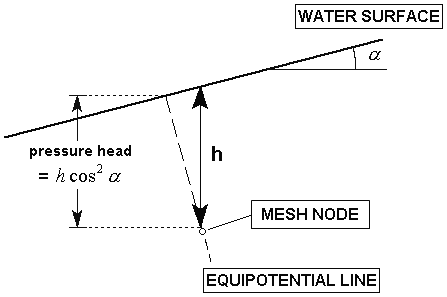Automatic Calculation of Hu coefficient

The Auto Hu option is a simple method of estimating pore pressures, based on the inclination of a Water Surface. In the absence of more accurate data (e.g. Seepage Analysis results), this is a simple but useful method of approximating head loss due to seepage.

## Ru Value

An Ru value simply models the pore pressure as a fraction of the vertical earth pressure. The vertical earth pressure is estimated from the unit weight and height of each material directly above a given point. The Ru Value is normally between 0 and 1.

An Ru Value can be specified for a material under the following conditions:

• If the Groundwater Method = Piezometric Lines in Project Settings, and Piezo to Use = None in the Define Hydraulic Properties dialog, then you can enter an Ru value for the material.
• If the Groundwater Method = Water Pressure Grid in Project Settings, and Grid to Use = None in the Define Hydraulic Properties dialog, then you can enter an Ru value for the material.

If one soil type has regions of differing Ru values, then a different material will have to be defined for each different Ru value. Appropriate material boundaries will have to be added to the model, in order to define the different soil regions.

## Grid to Use

If you are using water pressure grids to define pore pressure, the Grid to Use option allows you to select a water pressure grid to use for a material.

• If you have multiple grids defined, choose the name of the desired grid.
• If you want to specify zero pore pressure for the material then select Grid to Use = None.
• If Grid to Use = None, you can specify an Ru value to calculate pore pressure for the material (see above).
• If you want to stage pore pressure using multiple grids, then select the Stage Grids check box (see below).

## Stage Grids

If you have multiple water pressure grids defined, and you want to apply different grids at different stages, select the Stage Grids check box, and define the staging of the grids.

The Stage Grids option works in exactly the same way as the Stage Piezo Lines option described above.

## Copy To

The Copy To option allows you to define the groundwater/hydraulic information for one material, and then automatically copy the same information, to any other materials in the model. This is useful when all or most of the materials in a model, have the same groundwater conditions (for example, they all use the same piezo line).

The Copy To option is particularly useful if you are using the Stage Piezo Lines or Stage Grids options. The staging information can be defined once, and copied to other materials. For example:

• Define your Piezometric Line staging information for ONE material.
• Select Copy To. In the Copy Material Properties dialog, select the materials to which you would like to copy the information, and select OK.
• The Copy To option also copies the Hu value for the current material, to the selected materials.

## Undrained Behavior

RS2 offers an undrained effective stress analysis for soil layers in combination with effective strength parameters. RS2 uses the effective stress and strength and stress parameters to obtain the undrained shear strength of a material. Pore pressure development is critical in identifying the correct effective stress path which leads to a realistic failure value of undrained shear strength (cu or su).

#### 1. Undrained Effective Stress Calculation

When the undrained behaviour is selected for the material, special elements developed in RS2 will be automatically selected to account for the numerical stability when dealing with incompressible material.

Pore pressure in a soil body can usually be attributed to the presence of water and contributes to the total stress level within the soil. Terzaghi’s principle (see also 1.5) states that total stresses (σ) are divided into effective stresses (σ’), pore pressure (p), and pore water pressure (pw). Note that water does not sustain shear stress, therefore total shear stress is equal to effective shear stress.

σ = σ’ + mp (1.1a)

Where,

p = αSepw (1.1b)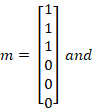σxx = σ’xx + αSep w (1.1c)

σyy = σ’yy + αSep w (1.1d)

σzz = σ’zz + αSep w (1.1e)

σxy = σ’xy (1.1f)

σyz = σ’yz (1.1g)

σzx = σ’zx (1.1h)

Where α = Biot’s pore pressure coefficient and Se = effective degree of saturation. For incompressible grains, Biot’s pore pressure coefficient becomes α = 1. Additional details about compressible grains and compressible solid material (α < 1) are provided in the Biot’s coefficient section below.

Pore pressure is the product αSepw and is termed p in RS2. An additional distinction must be made between steady state pore stress (psteady) and excess pore stress (pexcess).

pw = psteady + pexcess (1.2)

Steady state pore pressures (psteady) are determined based on phreatic levels or by a groundwater flow calculation; this is considered input data. During plastic calculations, excess pore pressures are generated for undrained material behaviour or during a consolidation analysis. The following equations can be used to describe undrained material behaviour and related excess pore pressure calculations:

The time derivative of the steady state component is equal to zero, therefore: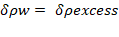(1.3)

Inverting Hooke’s law gives the following:

Substituting in Equation 1.1 gives the following:

In cases with slightly compressible water, excess pore pressure can be defined using the following: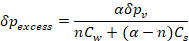(1.6a)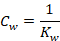(1.6b)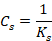(1.6c)

Kw represents the bulk modulus of the water, Ks represents the bulk modulus of the solid material, Cw represents the compressibility of the water, Cs is the compressibility of the solid material, and n is the porosity of the soil.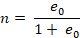(1.7)

e0 is the initial void ratio, as defined in the soil properties. The inverted form of Hooke’s law may be written in terms of the total stress rates and the undrained parameters Eu and vu: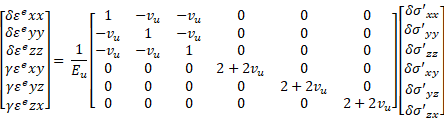(1.8)

Where,

where B represents Skempton’s B-parameter.

In RS2 undrained models, the effective parameters G and v’ are converted into their undrained counterparts Eu and Vu (see Equation 1.9 above).

To obtain realistic results, the bulk modulus of the water must be higher than the bulk modulus of the soil skeleton (Kw >> nK’). This can be achieved by requiring that v’ ≤ 0.35.

#### 2. Skempton B-Parameter

When using undrained drainage conditions, RS2 employs an implicit undrained bulk modulus (Ku) for the soil in its entirety (soil skeleton and water). The following equations are used to differentiate between total stress rates, effective stress rates, and rates of excess pore pressure:

Total stress: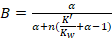(2.1a)

Excess pore pressure: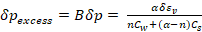(2.1b)

Effective stress: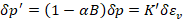(2.1c)

Undrained effective stiffness parameters should be entered with material properties (define E’ and v’, not Eu and Vu). Hooke’s law of elasticity is used to automatically calculate the undrained bulk modulus: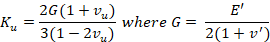(2.2)

When the user manually inputs Skempton’s B-parameter and Biot’s pore pressure coefficient, vu is calculated using the following equation: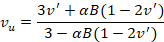(2.3)

The value of Skempton’s B-parameter is calculated using the ratio of the bulk stiffnesses of the soil skeleton and the pore fluid: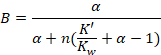(2.4)

Where Kw = 2 * 106 kN/m2.

The following equation is used to calculate the rate of excess pore pressure using the (small) volumetric strain rate: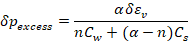(2.5)

The special undrained behaviour option in RS2 requires calculations that using effective stiffness parameters, with a distinction between effective stresses and excess pore pressures. The calculations may not completely address shear induced effective pore pressures.

This analysis requires effective soil parameters and is therefore very useful when these parameters are available. However, with soft soil projects, accurate effective soil parameter data may not be accessible. Therefore, in situ and laboratory tests may be performed to obtain undrained soil parameter data. In these cases, Hooke’s law can be used to convert measured values of undrained Young’s moduli into effective Young’s moduli: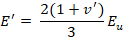(2.6)

This direct conversion from measured to effective values is not possible for advanced models. In these advanced cases, it is suggested to estimate the effective stress parameter from the measured parameter, then perform an undrained test to verify the resulting undrained stiffness (and adapt the estimated effective stiffness value if needed). The Soil test facility (Reference Manual) may be used in these circumstances.

#### 3. Biot Pore Pressure Coefficient, α

Generally, the compressibility of the soil skeleton will be greater than that of the individual grains of soil in a mass; therefore, deformations of individual soil grains can be disregarded. In cases with deep soil layers at high pressures, the stiffness of the soil/rock matrix will approach that of the material of the soil/rock grains; in these cases, the compressibility of the solid material must be considered. This affects the division of total stress into effective stress and pore pressure. In cases with compressible solid material, Terzaghi’s effective stress can be defined using the following equation: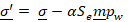(3.1)

Where α is Biot’s pore pressure coefficient, Se is the effective degree of saturation, m is a vector (with unity values for normal components and 0-values for shear components), and pw is the pore water pressure. The following equation shows the definition of Biot’s pore pressure coefficient: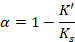(3.2)

Where K’ is the effective bulk modulus for the soil matrix and Ks is the bulk modulus of the solid material. Note that for an incompressible solid material (Ks = ∞), Terzaghi’s original stress definition holds true. Lower values of α indicate that for given values of total stress and pore water pressure, the resulting effective stress is higher than that of an incompressible solid material (α = 1).

The value of Biot’s pore pressure coefficient is automatically calculated by RS2, but the value may be changed manually by the user.

The following information for undrained behavior in RS2 can be found here.